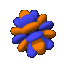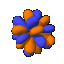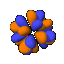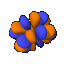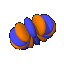# Petty Orbital Table

This is an abridgement of the
original table by Manthey

All atomic orbitals with n<=7 are presented here. Note that the orbitals with negative m are identical to those with the same magnitude positive m value except for a rotation,and are not shown separately. With the exception of the 1s0 orbital, all s0 orbitals are shown as cutaways to show the concentric spheres.

## n and l versus m

 m=0 m=1 m=2 m=3 m=4 m=5 m=6 n=1l=0(1s)n=2l=0(2s)n=2l=1(2p)n=3l=0(3s)n=3l=1(3p)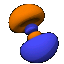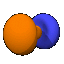n=3l=2(3d)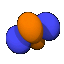n=4l=0(4s)n=4l=1(4p)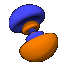n=4l=2(4d)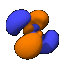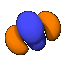n=4l=3(4f)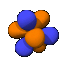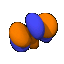n=5l=0(5s)n=5l=1(5p)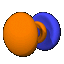n=5l=2>(5d)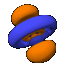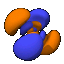n=5l=3(5f)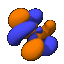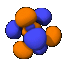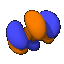n=5l=4(5g)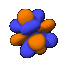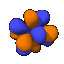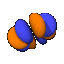n=6l=0(6s)n=6l=1(6p)n=6l=2(6d)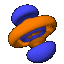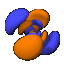n=6l=3(6f)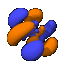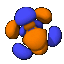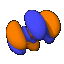n=6l=4(6g)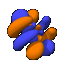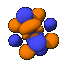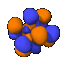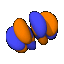n=6l=5(6h)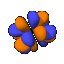n=7l=0(7s)n=7l=1(7p)n=7l=2(7d)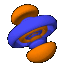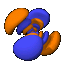n=7l=3(7f)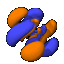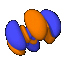n=7l=4(7g)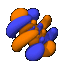n=7l=5(7h)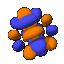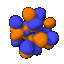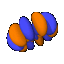n=7l=6(7i)